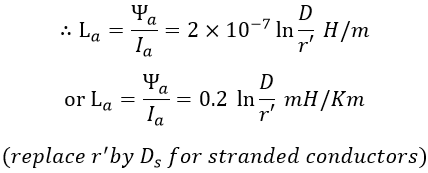# Inductance of Transmission Line - Single Phase & Three Phase

When the conductors of the transmission line carry alternating current, an alternating magnetic flux will be set up by the conductors. Due to the alternating nature of current, the flux linkages with the conductor change and hence causes inductance (induced emf) to be present in the conductor. The inductance of a conductor is given by the ratio of total magnetic flux linkages to the current flowing through it.

The total magnetic flux linkage is flux linkage to a conductor due to its own current and due to current in the neighboring conductors. Let us see the expressions for the inductance of a conductor in single-phase and three-phase transmission lines.

## Inductance of Singe-Phase Transmission Line :

Consider a single-phase overhead transmission line consisting of conductors A and B of radius r, spaced at a distance D apart as shown in the figure below. Let the conductors carry equal currents but as one conductor is 'go' and another is 'return', the sum of the currents is zero.

The total flux linkage of any conductor (say A) is due to its own current, called the internal flux linkage, and the current in the adjacent conductor, called the external flux linkage.

### Inductance of a Conductor due to Internal Flux :

Concentrating on conductor A of the above figure. Consider a point P at a radial distance of x m from the center of the conductor A. Such that x < R as shown below.

According to Ampere's Circuital Law, the field strength H at a radial distance x from the center of the conductor is given by,

H = Current enclosed by the region/(2π × Distance) At/m

### Inductance of a Conductor due to External Flux :

Consider the two points Q and R lying at a distance of R1, R2 respectively from the center of the conductor A as shown in the figure below.

According to Ampere's Circuital Law, the field strength at a point P distance x from the center of the conductor A such that x > r is given by,

H = 1/2πx AT/m

### Inductance of a Single-Phase Transmission Line :

We know that the flux linkage of a conductor is the sum of the internal and external flux linkages. Therefore the flux linkage of conductor A due to its own current is,

## Inductance of Three-Phase Transmission Line with Symmetrical Spacing :

Consider a 3-phase overhead transmission line with phase conductors a,b,c and are symmetrically spaced i.e., conductors are placed equidistant from each other as shown below. Let D be the distance between the conductors and r be the radius of each conductor.

Let Ia, Ib, and Ic be the currents of conductors a, b, and c. If the currents are assumed to be balanced, then,

Ia + Ib + Ic = 0
Ib + Ic = -Ia

#### The flux linkage of conductor 'a' due to currents Ia, Ib and Ic is given by,Therefore, the inductance of conductor 'a' is given as,Since the conductors are symmetrically spaced, the inductance per conductor is same for all the conductors. Hence, the inductance of conductors b and c is equal to the inductance of conductor a when they are symmetrically spaced.

Do not enter any spam links and messages